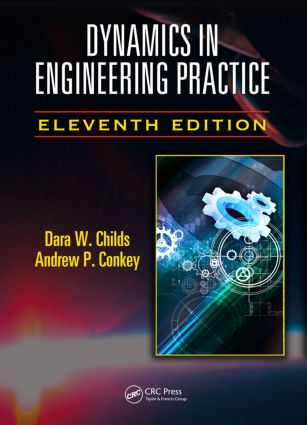Dynamics in Engineering Practice

11th Edition

CRC Press

474 pages | 706 B/W Illus.

Hardback: 9781482250251
pub: 2015-04-01
\$165.00
x
eBook (VitalSource) : 9780429162107
pub: 2015-04-17
from \$82.50

FREE Standard Shipping!

Description

Observing that most books on engineering dynamics left students lacking and failing to grasp the general nature of dynamics in engineering practice, the authors of Dynamics in Engineering Practice, Eleventh Edition focused their efforts on remedying the problem. This text shows readers how to develop and analyze models to predict motion. While establishing dynamics as an evolution of continuous motion, it offers a brief history of dynamics, discusses the SI and US customary unit systems, and combines topics that are typically covered in an introductory and intermediate, or possibly even an advanced dynamics course. It also contains plenty of computer example problems and enough tools to enable readers to fully grasp the subject. A free support book with worked computer examples using MATLAB® is available upon request.

New in the Eleventh Edition:

A large number of problems have been added; specifically, 59 new problems have been included in the original problem sets provided in chapters two through five. Chapter six has been added and covers the application of Lagrange’s equations for deriving equations of motion.

The new and improved chapters in this text:

• Address the fundamental requirements of dynamics, including units, force, and mass, and provides a brief history of the development of dynamics
• Explore the kinematics of a particle, including displacement, velocity, and acceleration in one and two dimensions
• Cover planar kinetics of rigid bodies, starting with inertia properties and including the mass moment of inertia, the radius of gyration, and the parallel-axis formula
• Explain how to develop equations of motion for dynamics using Lagrange’s equations

Dynamics in Engineering Practice, Eleventh Edition shows readers how to develop general kinematic equations and EOMs, analyze systems, and set up and solve equations, using a revolutionary approach to modeling and analysis along with current computer techniques.

Reviews

"It is easy to identify students who learned dynamics from (previous editions) of this book…. They are confident, they approach new problems based on fundamental principles, they are not afraid of dynamics. The integrated, differential equations & fundamental principles based approach removes the dread from dynamics! No longer is there fear an uncertainty of picking the correct equation & guessing the correct special case… every problem can be methodically approached from the same few principles and conquered."

—James R Morgan, Charles Sturt University, Bathurst, NSW, Australia

Introduction and Fundamentals

Introduction

Short History of Dynamics

Units

Planar Kinematics of Particles

Introduction

Motion in a Straight Line

Particle Motion in a Plane: Cartesian Coordinates

Coordinate Transformations: Relationships between Components of a Vector in Two-Coordinate Systems

Particle Motion in a Plane: Polar Coordinates

Particle Motion in a Plane: Normal-Tangential (Path) Coordinates

Moving between Cartesian, Polar-, and Path-Coordinate Definitions for Velocity and Acceleration Components

Time-Derivative Relationships in Two-Coordinate Systems

Velocity and Acceleration Relationships in Two Cartesian Coordinate Systems

Relative Position, Velocity, and Acceleration Vectors between Two Points in the Same Coordinate System

Summary and Discussion

Problems

Planar Kinetics of Particles

Introduction

Differential Equations of Motion for a Particle Moving in a Straight Line: An Introduction to Physical Modeling

More Motion in a Straight Line: Degrees of Freedom and Equations of Kinematic Constraints

Motion in a Plane: Equations of Motion and Forces of Constraint

Particle Kinetics Examples with More than 1DOF

Work-Energy Applications for 1DOF Problems in Plane Motion

Linear-Momentum Applications in Plane Motion

Moment of Momentum

Summary and Discussion

Problems

Planar Kinematics of Rigid Bodies

Introduction

Velocity and Acceleration Relationships for Two Points in a Rigid Body

Rolling without Slipping

Planar Mechanisms

Summary and Discussion

Problems

Planar Kinetics of Rigid Bodies

Introduction

Inertia Properties and the Parallel-Axis Formula

Governing Force and Moment Equations for a Rigid Body

Kinetic Energy for Planar Motion of a Rigid Body

Fixed-Axis-Rotation Applications of the Force, Moment, and Energy Equations

Compound Pendulum Applications

General Applications of Force, Moment, and Energy Equations for Planar Motion of a Rigid Body

Moment of Momentum for Planar Motion

Summary and Discussion

Problems

Lagrange’s Equations of Motion

Introduction

Deriving Lagrange’s Equations of Motion

Applying Lagrange’s Equation of Motion to Problems without Kinematic Constraints

Conservation of Momenta from Lagrange’s Equations of Motion

Application of Lagrange’s Equations to Examples with Algebraic Kinematic Constraints

Using Lagrange Multipliers to Define Reaction Forces for Systems with Generalized Coordinates

Summary and Discussion

Problems

A: Essentials of Matrix Algebra

B: Essentials of Differential Equations

Mass Properties of Common Solid Bodies

References

Dr. Dara Childs is professor of mechanical engineering at Texas A&M University (TAMU) in College Station, Texas. He has been director of the TAMU Turbomachinery Laboratory since 1984. He has received several best-paper awards, is an American Society of Mechanical Engineers (ASME) life fellow, and received the ASME Henry R. Worthington medal for outstanding contributions in pumping machinery. He is the author of many conference and journal papers plus two prior books. Dr. Childs has taught graduate and undergraduate courses in dynamics and vibrations since 1968: Colorado State University (1968–1971), University of Louisville (1971–1980), TAMU (1980–present).

Andrew P. Conkey received his PhD from Texas A&M University (TAMU) in 2007, where his research was in the application of the fiber Fabry–Perot interferometer to machinery/vibration measurements. He received his bachelor’s and master’s degrees from TAMU–Kingsville. He has over 16 years of teaching experience, having taught at TAMU–Kingsville, TAMU–College Station, TAMU–Qatar, and TAMU–Corpus Christi. In addition to teaching, he has worked for a refinery, a fiber-optic sensor company, and an engineering consulting firm.